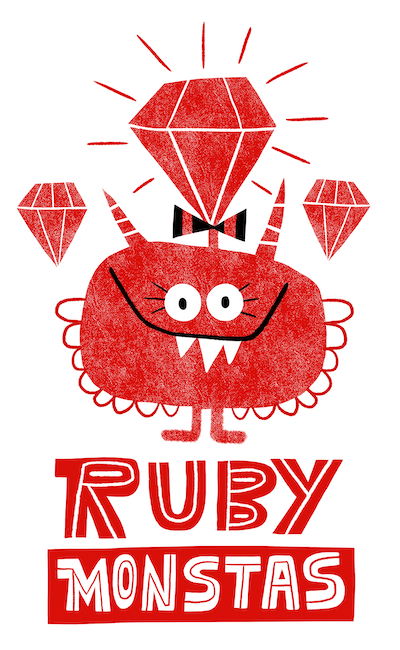# Ruby Monstas

Comparison Operators## Comparison Operators

Comparison operators are used to compare two things, typically numbers (integers or floats)

## Comparison Operators

Try these in IRB!

`5 == 4`
`=> false`
`5 != 4`
`=> true`
`1 < 2`
`=> true`

## Comparison Operators

Try these in IRB!

`1 > 2`
`=> false`
`7.1 <= 7.2`
`=> true`
`7.1 >= 7.2`
`=> false`

## Syntax

Syntax:
`expression1 operator expression2`
For example
`my_string.length > maximum_length`

Note: Expressions can contain variables and method calls, as we have seen before

Note: Comparison operators are like a yes/no question, therefore they always return either
`true`
or
`false`

## Overview

 `==` Are the left and right side equal? `!=` Are the left and right side not equal? `<` Is the left side smaller than the right side? `>` Is the left side bigger than the right side? `<=` Is the left side smaller than or equal to the right side? `>=` Is the left side bigger than or equal to the right side?

What questions do you have?